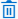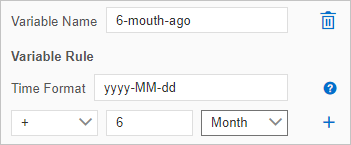This topic describes the variables that are involved in task orchestration and shows you how to configure time variables.

## Overview

When you configure a task node in a task flow, you can configure the following variables on the Variable Setting tab based on the node type:
• Node variables are time variables that can be used only on the current node.
• Task flow variables are time variables that can be used on all nodes of the current task flow.
Note You can configure task flow variables on one of the task nodes in a task flow. Then, the configuration of task flow variables is synchronized to other task nodes in the task flow.
• Input variables are automatically obtained by Data Management (DMS). You can use \${var_name} to reference an input variable in SQL statements for the current node. You can also use an input variable as a filter condition for a Conditional Branch node. The following variables are considered as input variables:
• Upstream variables are output variables from upstream nodes.
• Status variables: For more information, see the Status variables section of this topic.
• System variables: For more information, see the System variables section of this topic.
• Output variables are defined and have their values assigned on the current node. Output variables can be accessed and referenced by downstream nodes.

For example, if you configure an output variable on a Script node, the output variable can be referenced in the SQL statements of a downstream node.

• Script output variables: If the last line of the script file is a JSON string in the format of `{ key1: value1, key2: value2, … }` for a Script node, the script task parses the key-value pairs in the JSON string to obtain output variables. For each variable, the name is a key and the value is the value of the key. You can reference a script output variable in the format of `\${key}` in the SQL statements of a downstream node.

For example, if the last line of the script file is `echo {"hello": "world"}`, the script task parses the key-value pair to obtain an output variable whose name is hello and value is world.

## Configure time variables

Parameter Description
Variable Name The name of the custom time variable.
Note To delete a time variable that you configured, click theicon to the right of the Variable Name field.
Variable Rule The time format and time offset configurations of the custom time variable.
• Time Format: the time format of the time variable. For more information, see the Time formats section of this topic.
• Time offset: Time variables are offset from the data timestamp, which is one day before the current date. In other words, the value of a custom time variable indicates the date to which the specified time offset points based on the data timestamp.

For example, you have created a variable named `6_month_ago` in the yyyy-MM-dd format and set the offset to "- 6 Month". In this case, if the current date is August 12, 2021, the value of the `\${6_month_ago}` variable is 2021-02-11, which indicates February 11, 2021.Note After you configure a time variable, you can reference the variable in the `\${var_name}` format in SQL statements for the current node. You can also click SQLPreview to view the value of the time variable.

## Time formats

The following table describes the time variables that can be used to define the time format of a custom variable.

Time variable Description Sample format Sample value
Year
• y or yyyy in the four-digit format indicates the year of the day when the task is run.
• yy indicates the last two digits of the year.
• Y: the year of the last day in the week when the task is run. The last day of the week is Sunday.
yyyy 2021
Month M: the month of the year when the task is run. Valid values of M: [1,12]. Valid values of MM: [01,12]. MM 08
Week
• w: the week of the year when the task is run. Valid values of w: [1,52]. Valid values of ww: [01,52].
• W: the week of the month when the task is run. Valid values: [1,5].
ww 13
Day
• D: the day of the year when the task is run. Valid values of D: [1,365]. Valid values of DD: [01,365]. Valid values of DDD: [001,365].
• d: the day of the month when the task is run. Valid values of d: [1,31]. Valid values of dd: [01,31].
D 360
Day of the week
• E: the day of the week when the task is run. Valid values: Monday to Sunday.
• e: the number of the day in the week when the task is run. Valid values: [1,7]. A value of 1 indicates Monday.
Note To count Sunday as the first day of the week, you can set the offset to "+ 1 Day".
e 1
Ante meridiem (AM) or post meridiem (PM) a: indicates whether the point in time when the task is run is before or after the midday. If the point in time is in the range of 00:00 to 11:59, the return value is AM. If the point in time is in the range of 12:00 to 23:59, the return value is PM. a AM
Hour
• H: the hour of the day when the task is run. A value of 0 indicates the first hour of the day. Valid values of H: [0,23]. Valid values of HH: [00,23].
• h: the hour of the half day when the task is run. A value of 1 indicates the first hour of the half day. Valid values of h: [1,12]. Valid values of hh: [01,12].
• K: the hour of the half day when the task is run. A value of 0 indicates the first hour of the half day. Valid values of K: [0,11]. Valid values of KK: [00,11].
• k: the hour of the day when the task is run. A value of 1 indicates the first hour of the day. Valid values of k: [1,24]. Valid values of kk: [01,24].
HH 10
Minute m: the minute of the hour when the task is run. Valid values of m: [0,59]. Valid values of mm: [00,59]. m 27
Second
• s: the second of the minute when the task is run.
• S: the millisecond of the minute when the task is run.
ss 08
Time zone z: the time zone. z UTC+08:00

The following table describes the sample time formats that use multiple time variables.

Sample format Sample value
`yyyy-MM-dd` 2021-08-12
`yyyyMM01` 20210801
`HH:mm:ss` 11:05:21
`yyyyMMdd HH:mm:ss` 20210812 11:05:21

## Status variables

Variable Description
all_success All of the tasks are successfully run.
all_failed All of the tasks fail to be run.
one_failed A task of the current task flow fails to be run.
Note You can use status variables on a Conditional Branch node to make a conditional evaluation in a task flow. The subsequent tasks in the task flow can be run only if the Conditional Branch node meets the specified conditions.

## System variables

Variable Description Example
sys.flow.start.timestamp The timestamp generated when the task is run. 2021-05-24T11:20:07.562+08:00
sys.flow.start.year The year when the task is run. 2021
sys.flow.start.month The month of the year when the task is run. 5
sys.flow.start.day The day of the month when the task is run. 24
sys.flow.start.hour The hour of the day when the task is run. 11
sys.flow.start.minute The minute of the hour when the task is run. 20
sys.flow.start.second The second of the minute when the task is run. 7
sys.flow.start.milliseconds The millisecond of the second when the task is run. 562
sys.flow.start.timezone The time zone. Asia/Shanghai
sys.flow.biztime The data timestamp. By default, the data timestamp is the previous day of the day when the task is run. 1621740007562
sys.flow.name The name of the task flow. dwd_activityDailyPV
sys.node.name The name of the task. Single Instance SQL-1